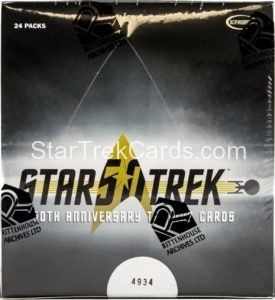Box Details
Box Location
Star Trek: Mixed Series → Star Trek 50th Anniversary → Other Items
Front Middle / Other BackTitle Number Number Made Manufacturer Material Size
Box of 24 Packs None Unknown Rittenhouse Archives Cardboard / Paper Unknown
Year Original Art Autograph Costume / Relic Sticker Die Cut
2016 No No No No No
Notes
None

Non-Graded Price History Averages
1 Month 3 Months 6 Months 1 Year 3 Years Lifetime
[insert_php] \$counter = 0; \$price=0; [/insert_php][insert_php] if(strtotime('April 11, 2017')>strtotime('-1 months')){ \$counter++; \$price = \$price + 64.10; } [/insert_php][insert_php] if(strtotime('April 13, 2017')>strtotime('-1 months')){ \$counter++; \$price = \$price + 71.95; } [/insert_php][insert_php]; if (\$counter > 0) { //checks to make sure there won't be a divide by zero error \$totalaverage = (\$price / \$counter); //divide monetary sum by the number of entries if (\$_GET['item_number'] != \$_GET['reference']) { \$totalaverage = (\$totalaverage / \$_GET['num']); } } echo '\$'; //echo the dollar sign round (\$totalaverage, 2); //round the number to a maximum of two decimal places echo number_format((float)\$totalaverage, 2, '.', ''); //force two decimal places for monetary consistency [/insert_php] [insert_php] \$counter = 0; \$price=0; [/insert_php][insert_php] if(strtotime('April 11, 2017')>strtotime('-3 months')){ \$counter++; \$price = \$price + 64.10; } [/insert_php][insert_php] if(strtotime('April 13, 2017')>strtotime('-3 months')){ \$counter++; \$price = \$price + 71.95; } [/insert_php][insert_php]; if (\$counter > 0) { //checks to make sure there won't be a divide by zero error \$totalaverage = (\$price / \$counter); //divide monetary sum by the number of entries if (\$_GET['item_number'] != \$_GET['reference']) { \$totalaverage = (\$totalaverage / \$_GET['num']); } } echo '\$'; //echo the dollar sign round (\$totalaverage, 2); //round the number to a maximum of two decimal places echo number_format((float)\$totalaverage, 2, '.', ''); //force two decimal places for monetary consistency [/insert_php] [insert_php] \$counter = 0; \$price=0; [/insert_php][insert_php] if(strtotime('April 11, 2017')>strtotime('-6 months')){ \$counter++; \$price = \$price + 64.10; } [/insert_php][insert_php] if(strtotime('April 13, 2017')>strtotime('-6 months')){ \$counter++; \$price = \$price + 71.95; } [/insert_php][insert_php]; if (\$counter > 0) { //checks to make sure there won't be a divide by zero error \$totalaverage = (\$price / \$counter); //divide monetary sum by the number of entries if (\$_GET['item_number'] != \$_GET['reference']) { \$totalaverage = (\$totalaverage / \$_GET['num']); } } echo '\$'; //echo the dollar sign round (\$totalaverage, 2); //round the number to a maximum of two decimal places echo number_format((float)\$totalaverage, 2, '.', ''); //force two decimal places for monetary consistency [/insert_php] [insert_php] \$counter = 0; \$price=0; [/insert_php][insert_php] if(strtotime('April 11, 2017')>strtotime('-1 year')){ \$counter++; \$price = \$price + 64.10; } [/insert_php][insert_php] if(strtotime('April 13, 2017')>strtotime('-1 year')){ \$counter++; \$price = \$price + 71.95; } [/insert_php][insert_php]; if (\$counter > 0) { //checks to make sure there won't be a divide by zero error \$totalaverage = (\$price / \$counter); //divide monetary sum by the number of entries if (\$_GET['item_number'] != \$_GET['reference']) { \$totalaverage = (\$totalaverage / \$_GET['num']); } } echo '\$'; //echo the dollar sign round (\$totalaverage, 2); //round the number to a maximum of two decimal places echo number_format((float)\$totalaverage, 2, '.', ''); //force two decimal places for monetary consistency [/insert_php] [insert_php] \$counter = 0; \$price=0; [/insert_php][insert_php] if(strtotime('April 11, 2017')>strtotime('-3 years')){ \$counter++; \$price = \$price + 64.10; } [/insert_php][insert_php] if(strtotime('April 13, 2017')>strtotime('-3 years')){ \$counter++; \$price = \$price + 71.95; } [/insert_php][insert_php]; if (\$counter > 0) { //checks to make sure there won't be a divide by zero error \$totalaverage = (\$price / \$counter); //divide monetary sum by the number of entries if (\$_GET['item_number'] != \$_GET['reference']) { \$totalaverage = (\$totalaverage / \$_GET['num']); } } echo '\$'; //echo the dollar sign round (\$totalaverage, 2); //round the number to a maximum of two decimal places echo number_format((float)\$totalaverage, 2, '.', ''); //force two decimal places for monetary consistency [/insert_php] [insert_php] \$counter = 0; \$price=0; [/insert_php][insert_php] \$counter++; \$price = \$price + 64.10; [/insert_php][insert_php] \$counter++; \$price = \$price + 71.95; [/insert_php][insert_php]; if (\$counter > 0) { //checks to make sure there won't be a divide by zero error \$totalaverage = (\$price / \$counter); //divide monetary sum by the number of entries if (\$_GET['item_number'] != \$_GET['reference']) { \$totalaverage = (\$totalaverage / \$_GET['num']); } } echo '\$'; //echo the dollar sign round (\$totalaverage, 2); //round the number to a maximum of two decimal places echo number_format((float)\$totalaverage, 2, '.', ''); //force two decimal places for monetary consistency [/insert_php]

Price History Detail
Date Sold Price Graded Condition Location User
April 13, 2017 \$71.95 No Near Mint/Mint (7-10) Ebay admin
April 11, 2017 \$64.10 No Near Mint/Mint (7-10) Ebay admin